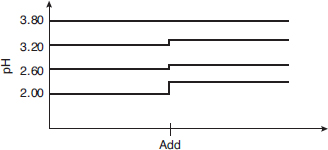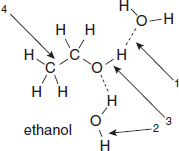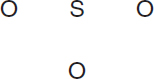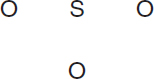# AP Chemistry Practice Test 33

### Test Information12 questions18 minutes

`Questions 1-3 refer to the following information.Four different 0.0100 M acid solutions were prepared, and their pH values were recorded on a laptop computer. One of the solutions contains more than just an acid. At the point designated as "add," all four solutions are diluted with an equal volume of water. The bottom line represents solution 1, and the top line represents solution 4.`

1. Using the data in the graph, which of the solutions does not contain just an acid dissolved in water?

2. Using the data presented in the graph and the experiment that was performed, which of the weak acids is the weakest?

3. Which of the acids will react with copper metal?

4. Chemists often ascribe the macroscopic properties of solids to the underlying microscopic structure. Silicon carbide is almost as hard and brittle as diamond. The solid state structure of silicon carbide is often described as

5.In the diagram above, which labeled arrow is pointing toward a covalent bond and which is pointing toward a hydrogen bond?

Covalent Bond     Hydrogen Bond

6. Which is the easiest way to burn a silver coin?

`Questions 7-10 refer to the following information.VesselABCGasArgonNeonXenonFormulaArNeXeMolar mass40 g/mol20 g/mol132 g/molPressure0.3 atm0.4 atm0.2 atmTemperature400 °C500 °C500 °CNote that in addition to the data in the table, the gases are held in separate, identical, rigid vessels.`

7. Which sample has the lowest density?

8. The average kinetic energy

9. Which of these gases is expected to condense at the lowest pressure, assuming that the temperature is held constant?

10. Which attractive force is the major cause of condensation of the three compounds in the table above?

11.Use the arrangement of atoms suggested in the skeleton structure above to construct the Lewis structure for the SO3 molecule. Which of the following statements about this molecule is incorrect?

12.Use the arrangement of atoms suggested in the skeleton structure above to construct the Lewis structure for the sulfite ion. Be sure to minimize the formal charges. Which statement is the least likely to be true?